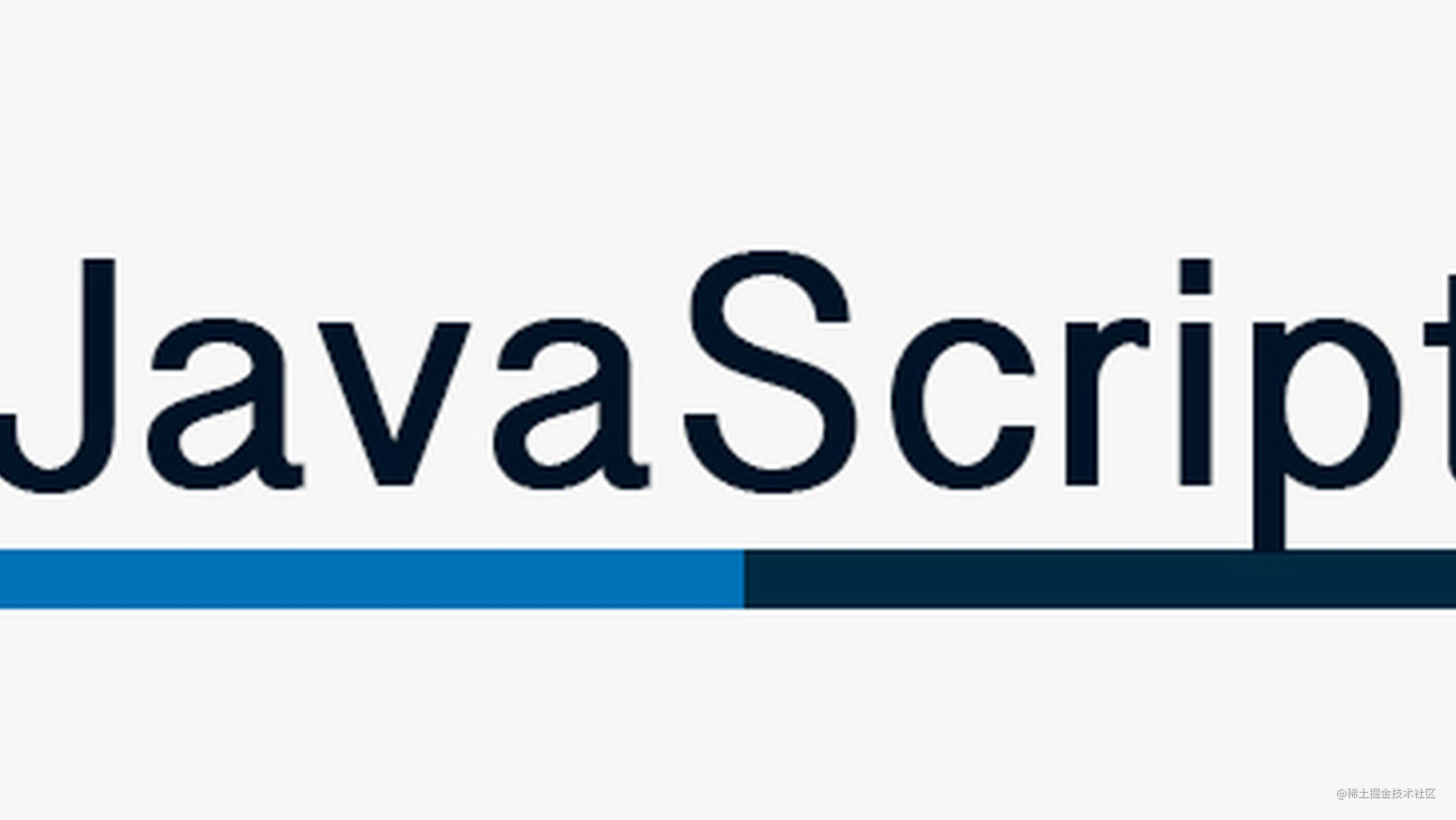# JavaScript 专题之函数记忆JavaScript 专题系列第十七篇，讲解函数记忆与菲波那切数列的实现

## 定义

``````function add(a, b) {
return a + b;
}

// 假设 memorize 可以实现函数记忆

memoizedAdd(1, 2) // 3
memoizedAdd(1, 2) // 相同的参数，第二次调用时，从缓存中取出数据，而非重新计算一次复制代码``````

## 第一版

``````// 第一版 (来自《JavaScript权威指南》)
function memoize(f) {
var cache = {};
return function(){
var key = arguments.length + Array.prototype.join.call(arguments, ",");
if (key in cache) {
return cache[key]
}
else return cache[key] = f.apply(this, arguments)
}
}复制代码``````

``````var add = function(a, b, c) {
return a + b + c
}

console.time('use memorize')
for(var i = 0; i < 100000; i++) {
}
console.timeEnd('use memorize')

console.time('not use memorize')
for(var i = 0; i < 100000; i++) {
}
console.timeEnd('not use memorize')复制代码``````

## 第二版

``````var propValue = function(obj){
return obj.value
}

var memoizedAdd = memorize(propValue)

console.log(memoizedAdd({value: 1})) // 1
console.log(memoizedAdd({value: 2})) // 1复制代码``````

``````// 第二版 (来自 underscore 的实现)
var memorize = function(func, hasher) {
var memoize = function(key) {
var cache = memoize.cache;
var address = '' + (hasher ? hasher.apply(this, arguments) : key);
cache[address] = func.apply(this, arguments);
}
};
memoize.cache = {};
return memoize;
};复制代码``````

``````var add = function(a, b, c) {
return a + b + c
}

memoizedAdd(1, 2, 3) // 6
memoizedAdd(1, 2, 4) // 6复制代码``````

``````var memoizedAdd = memorize(add, function(){
var args = Array.prototype.slice.call(arguments)
return JSON.stringify(args)
})

console.log(memoizedAdd(1, 2, 3)) // 6
console.log(memoizedAdd(1, 2, 4)) // 7复制代码``````

## 适用场景

``````var count = 0;
var fibonacci = function(n){
count++;
return n < 2? n : fibonacci(n-1) + fibonacci(n-2);
};
for (var i = 0; i <= 10; i++){
fibonacci(i)
}

console.log(count) // 453复制代码``````

``````当执行 fib(0) 时，调用 1 次

``````var count = 0;
var fibonacci = function(n) {
count++;
return n < 2 ? n : fibonacci(n - 1) + fibonacci(n - 2);
};

fibonacci = memorize(fibonacci)

for (var i = 0; i <= 10; i++) {
fibonacci(i)
}

console.log(count) // 12复制代码``````

## 专题系列

JavaScript专题系列目录地址：github.com/mqyqingfeng…

JavaScript专题系列预计写二十篇左右，主要研究日常开发中一些功能点的实现，比如防抖、节流、去重、类型判断、拷贝、最值、扁平、柯里、递归、乱序、排序等，特点是研(chao)究(xi) underscore 和 jQuery 的实现方式。